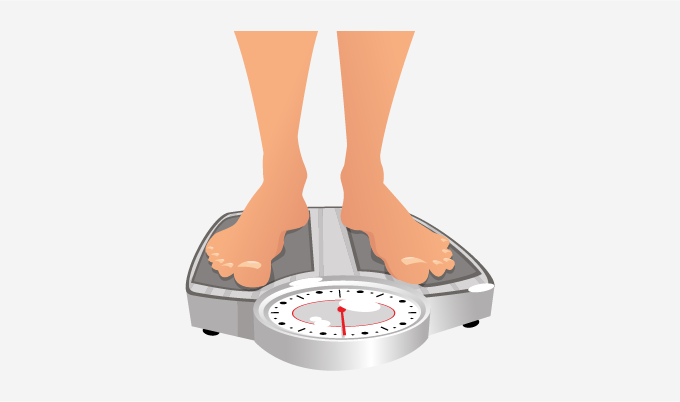Rounding Decimals - Definition with Examples

The Complete K-5 Math Learning Program Built for Your Child

• 30 Million Kids

Loved by kids and parent worldwide

• 50,000 Schools

Trusted by teachers across schools

• Comprehensive Curriculum

Aligned to Common Core

Rounding Decimals

Rounding is a process to estimate a particular number in a context. To round a number look at the next digit in the right place, if the digit is less than 5, round down and if the digit is 5 or more than 5, round up.

Rounding decimals

Rounding decimals refer to the rounding of decimal numbers to a certain degree of accuracy. We can round decimals to the nearest wholes, tenths or hundredths.

Rounding decimals is useful to estimate an answer easily and quickly. It is also useful to find out the estimate of the average score of the students in a class.

1. Rounding to the nearest whole

We follow the given steps to round numbers to the nearest whole number:

Step 1- We look at the number we want to round.

Step 2- As we are rounding our number to the nearest whole, we mark the digit in the one's place.

Step 3- Now we look at the ‘tenths’ place (the digit to the right of the decimal point).

Step 4- (i) If the digit in the tenths column is 0, 1, 2, 3 or 4, we will round down the number at the ones place to the nearest whole number.

(ii) If the digit in the tenths column is 5, 6, 7, 8 or 9, we will round up the number at the one's place to the nearest whole number.

Step 5- Remove all the digits after the decimal point. The left out number is the desired answer.

Example 1: Round 945.65 to the nearest whole number.

 Step Number Observation Working Out Step 1 945.65 Step 2 We get 5 in the one's column. Step 3 We get 6 in the hundredths column (right of 5) Step 4 We get 6 in Step 3. Round-Up Step 5 946 We add 1 to 5 and remove all the digits from the right of the one's place.

2. Rounding to the nearest tenths

We follow the given steps to round numbers to the nearest tenths:

Step 1- We look at the number we want to round.

Step 2- As we are rounding our number to the nearest tenths, we mark the digit in the tenths place.

Step 3- Now we look at the ‘hundredths’ place (the digit to the right of the tenths column).

Step 4- (i) If the digit in the hundredths place is 0, 1, 2, 3 or 4, we will round down the number at the tenths place to the nearest tenths.

(ii) If the digit in the hundredths place is 5, 6, 7, 8 or 9, we will round up the number at the tenths place to the nearest tenths.

Step 5- Remove all the digits to the right of the tenths column. The left out number is the answer.

Example 2: Round 542.33 to the nearest tenths.Step Number Observation Working Out Step 1 542.33 Step 2 We get 3 in the tenths column. Step 3 We get 3 in the hundredths place (right of 3) Step 4 We get 3 in Step 3. Round-Down Step 5 542.3 We keep 3 as it is, and remove all the digits from the right of the tenths column.

Example 3: Ryan weighs 27.51 kg. What is his weight to the nearest kg?

 Step Number Observation Working Out Step 1 27.51 Step 2 We get 7 in the one's place. Step 3 We get 5 in the tenths place (right of 7) Step 4 We get 5 in Step 3. Round-Up Step 5 28 We add 1 to 7 and remove all the digits from the right of the ones place.

The weight to the nearest kg = 28 kg.3. Rounding to the nearest hundredths

We can follow the given steps to round numbers to the nearest hundredths:

Step 1- We look at the number we want to round.

Step 2- As we are rounding our number to the nearest hundredths, we mark the digit in the hundredths place.

Step 3- Now we look at the ‘thousandths’ place (the digit to the right of the hundredths column).

Step 4- (i) If the digit in the thousandths place is 0, 1, 2, 3 or 4, we will round down the hundredths place to the nearest hundredths.

(ii) If the digit in the thousandths column is 5, 6, 7, 8 or 9, we will round up the hundredths place to the nearest hundredths.

Step 5- Remove all the digits to the right of the hundredths place. The left out number is the answer.

Example 4: The depth of lake Tanganyika is 1,470.158 m. What is the depth of the lake to the nearest hundredths?

 Step Number Observation Working Out Step 1 1470.158 Step 2 We get 5 in the hundredths place. Step 3 We get 8 in the thousandths place (right of 5) Step 4 We get 8 in Step 3. Round-Up Step 5 1470.16 We add 1 to 5, and remove all the digits from the right of the hundredths column.

 Fun Fact When we do rounding we get an approximate value. A special type of symbol is used to show the approximate value. For example 6.9 = 7

Won Numerous Awards & Honors